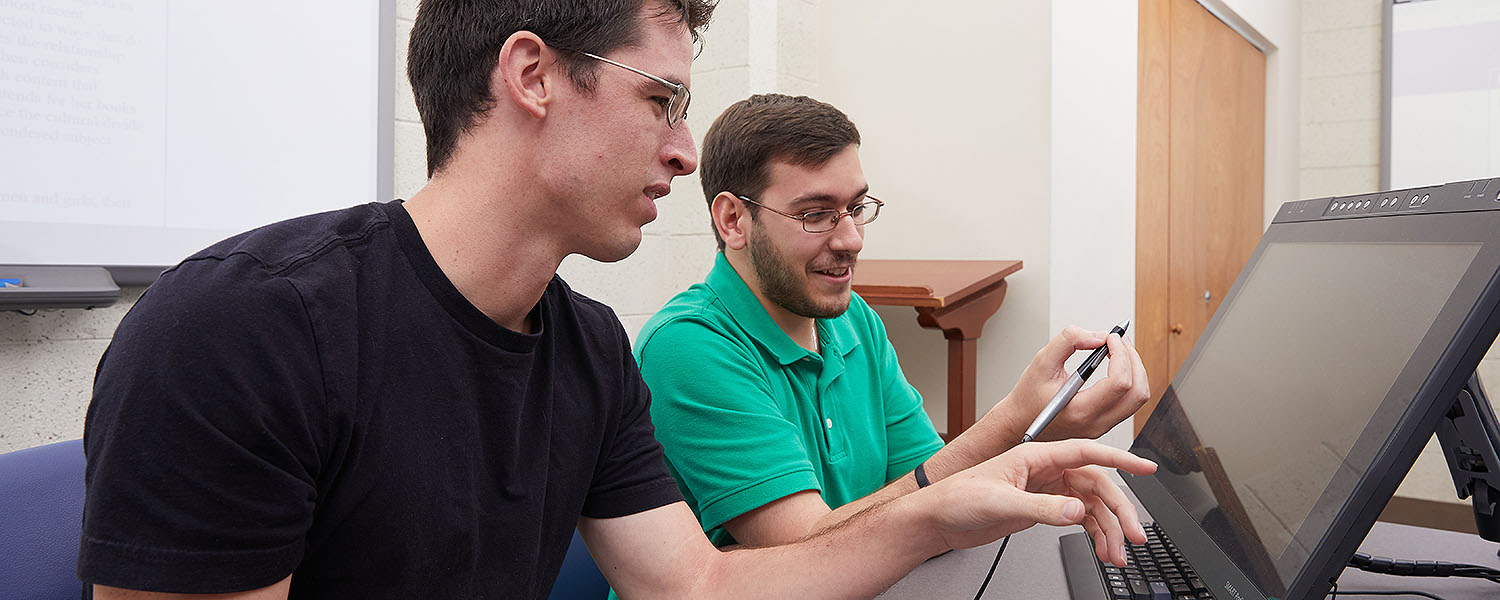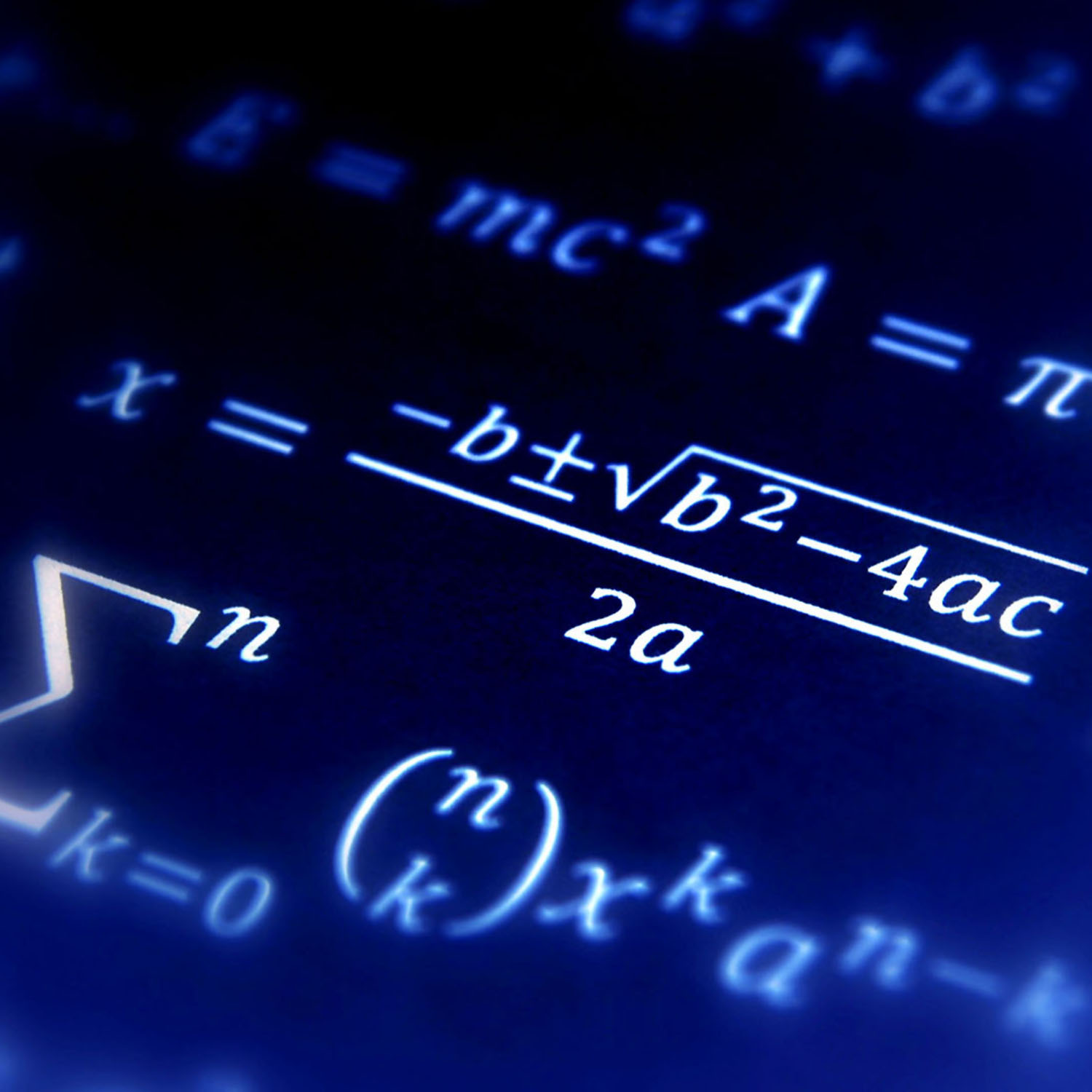# Research OverviewThe research activities of the faculty in the School of Mathematics and Statistical Sciences can be roughly divided into seven areas: algebra, discrete mathematics and number theory; pure and applied analysis; computational mathematics; mathematical biology; operations research; statistics and probability; and applied statistics and data science.

## Research Area Overview

The field of algebra, discrete mathematics and number theory encompasses one of the primary branches of pure mathematics. Problems in this field often arise — or follow naturally from — a problem that is easily stated involving counting, divisibility or some other basic arithmetic operation.

The study of pure and applied analysis provides a basic understanding of qualitative and quantitative problem-solving techniques, the ability to analyze new areas of interest, and the ability to interact with colleagues from other disciplines in a problem-solving situation. Modern applications of analysis include biomedical modeling, image analysis, robotic control, ecology, environmental modeling and financial engineering.Advanced work in all areas of science and technology relies critically on computation. Computational mathematics involves the design and analysis of mathematical models for various problems and the construction of algorithms which efficiently and accurately compute solutions.

Mathematical biology is a very diverse group, both in the biological systems studied and in mathematics used to study these systems. Biological applications include population dynamics, epidemiology of infectious diseases, biochemical networks and systems biology.

Operations research is distinguished by its use of quantitative methods (mathematics, statistics and computing) to aid in rational decision making.

Graduate study in statistics and probability has taken on a new look and increased importance in the last two decades due to dramatically increased computational power and the aggressive and highly successful application of statistical methods by competitors in the world marketplace.

Applied statisticians and data scientists collaborate with scientists in academia, industry and government on the design, implementation and analysis of research studies.

School of Mathematical and Statistical Sciences
School of Mathematical and Statistical Sciences | O-110 Martin Hall, Clemson, SC 29634